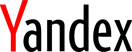(panoramic photo of Moscow from moscow.photobase.ru)# Eighth International Conference on Computability, Complexity and Randomness (CCR 2013)

### September 23–27, 2013, Moscow, Russia## Nonstandard models of arithmetic and Ramsey theorem

Yue Yang
n this talk, I will start with talking about two seemingly disjoint areas related to recursion theory. One is to study computability on weak fragments of arithmetic, whose models are necessarily nonstan- dard. The other is reverse mathematics, which asks what kind of axioms are needed in order to prove a mathematical theorem. With a few ex- ceptions, most of the results in reverse mathematics make use of the so-called “ω-model”, which has the standard model ω as its first order part. In the recent study of Ramsey Theorem for pairs, we discovered a nice application of nonstandard models to reverse mathematics. Using nonstandard models, we have obtained better understanding of the first order and second order consequences of combinatorial statements relat- ed to Ramsey theorem for pairs. This is a joint work with C.T. Chong and Ted Slaman.